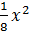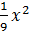### Sample Problem

What is the equation of a parabola with vertex at the origin and a directrix at y = -3?

(A) y =(B) y =(C) y =(D) y =#### Solution

y = -p

y = -3

p = 3

4(3)y = x2

12y = x2

y =